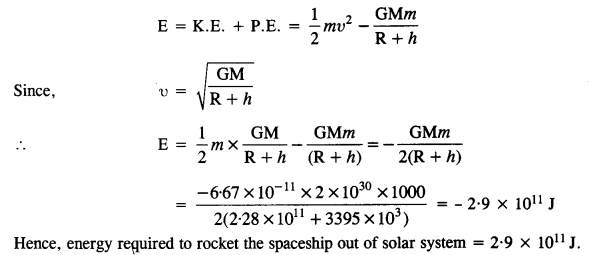## NCERT Solutions for Class 11 Physics Chapter 8 Gravitation

These Solutions are part of NCERT Solutions for Class 11 Physics. Here we have given NCERT Solutions for Class 11 Physics Chapter 8 Gravitation

Question 1.
(a) You can shield a charge from electrical forces by putting it inside a hollow conductor. Can you shield a body from the gravitational influence of nearby matter by putting it inside a hollow sphere or by some other means?
(b) An astronaut inside a small space ship orbiting around the earth cannot detect gravity. If the space station orbiting around the Earth has a large size, can he hope to detect gravity?
(c) If you compare the gravitational force on the Earth due to the Sun to that due to the moon, you would find that the Sun’s pull is greater than the moon’s pull (you can check this yourself using the data available in the succeeding exercises). However, the tidal effect of the moon’s pull is greater than the tidal effect of the Sun. Why?
(a) Gravitational force on a body inside a hollow sphere is zero. However, a gravitational force acts on a body inside a hollow sphere due to bodies lying outside the hollow sphere. Hence a body cannot be shielded from the gravitational influence of nearby matter.
(b) Yes, with a larger mass, the value of gravity will be significant inside the spaceship.
(c) Tidal effectAlthough Sun’s pull is more than the moon, yet tidal effect due to the moon is more because the moon is nearer to the earth.

Question 2.
Choose the correct alternative:
(a) Acceleration due to gravity increases/decreases with increasing altitude.
(b) Acceleration due to gravity increases/decreases with increasing depth (assume the Earth to be a sphere of uniform density).
(c) Acceleration due to gravity is independent of the mass of the Earth/mass of the body.
(d) The formula -G Mm (1/r2 – 1/r1) is more/less accurate than the formula mg (r2 – r1) for the difference of potential energy between two points r2 and r1 distance away from the center of the Earth.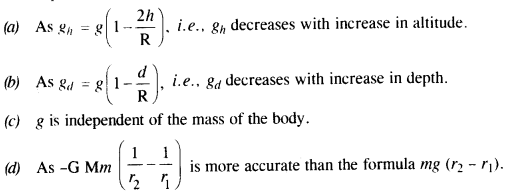Question 3.
Suppose there existed a planet that went around the sun twice as fast as the Earth. What would be its orbital size as compared to that of the Earth?Question 4.
One of the satellites of Jupiter has an orbital period of 1.769 days and the radius of the orbit is 4.22 x 108 m. Show that the mass of Jupiter is about one thousand times that of the sun. (Take 1 year = 365.25 mean solar day).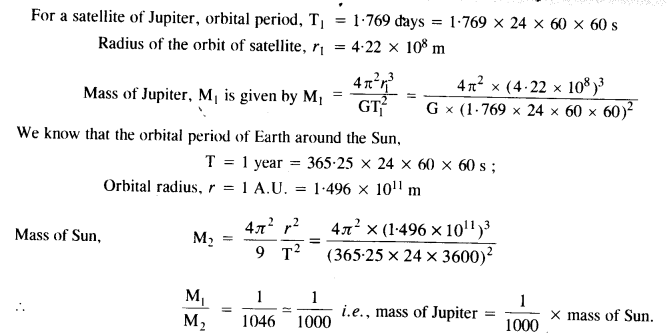Question 5.
Let us consider that our galaxy consists of 2.5 x 1011 stars each of one solar mass. How long will this star at a distance of 50,000 ly from the galactic center take to complete one revolution? Take the diameter of the Milky Way to be 105 ly. G = 6.67 x 1011 Nm2 kg2.
r = 50,000 ly = 50,000 x 9.46 x 1015m = 4.73 x 1020m
M = 2.5 x 1011 solar mass = 2.5 x 10n x (2 x 1030) kg
= 5.0 x 1041 kg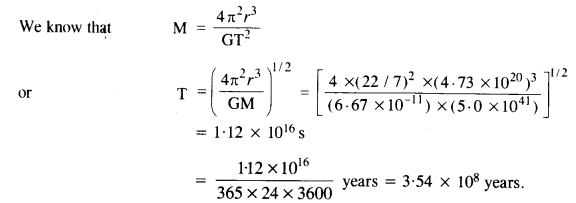Question 6.
Choose the correct alternative:
(1) If the zero of the potential energy is at infinity, the total energy of an orbiting satellite is negative of its kinetic/potential energy.
(2) The energy required to rocket an orbiting satellite out of earth’s gravitational influence is more/less than the energy required to project a stationary object at the same height (as the satellite) out of Earth’s influence.
1. Kinetic energy
The potential energy of a satellite rotating in its orbit is zero. The total energy of a system is the sum of its kinetic energy (+ve) and potential energy. Since the earth satellite system is a bound system. The satellite has negative total energy. So, the energy of the satellite is the negative of its kinetic energy.

2. Less
An orbiting satellite has more energy than a stationary object at the same height. This additional energy is provided by the orbit. It requires lesser energy to make it move out of the earth’s influence than a stationary object.

Question 7.
Does the escape speed of a body from the Earth depend on
(a) the mass of the body,
(b) the location from where it is projected,
(c) the direction of projection,
(d) the height of the location from where the body is launched? Explain your answer.
(a) Escape speed of a body is independent of the mass of a body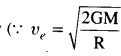where M is the mass of early
(b) Escape speed of a body depends upon the location.
(c) Escape speed of a body is independent of the direction of projection.
(d) Escape speed of a body depends upon the height of the location from where the body is projected because the escape velocity depends upon the gravitational potential at the point from which it is projected and this potential depends upon height also

Question 8.
A comet orbits the sun in a highly elliptical orbit. Does the comet have a constant
(a) linear speed,
(b) angular speed,
(c) angular momentum,
(d) kinetic energy,
(e) potential energy,
(f) total energy throughout its orbit?
Neglect any mass loss of the comet when it comes very close to the Sun.
Angular momentum and total energy do not vary throughout the orbit whereas the rest all the quantities vary in the orbit.

Question 9.
Which of the following symptoms is likely to afflict an astronaut in space :
(a) swollen feet,
(b) swollen face,
(d) orientational problem?
The astronaut in space will suffer from
(b) swollen face,
(d) orientational problem.

Question 10.
The gravitation intensity at the center of the drum head defined by a hemispherical shell has the direction indicated by the arrow (see Fig.), (i) a, (ii) b, (iii) c, (iv) zero.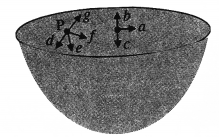Intensity inside a shell is zero, so it will be zero at P and Q also (Potential is constant).

Question 11.
For the above problem, the direction of the gravitational intensity at an arbitrary point P is indicated by the arrow (i) d, (ii)y, (iii) f, (iv) g.
As in the fig, upper portion of the shell is missing, so gravitational intensity at P and Q should act along e and c respectively.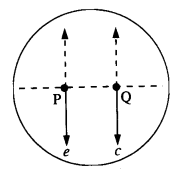Question 12.
A rocket is fired from the earth towards the Sun. At what distance from the Earth’s center is the gravitational force on the rocket zero? Mass of the Sun = 2 x 1030 kg, mass of the Earth = 6 x 1024 kg. Neglect the effect of other planets etc. (orbital radius = 1.5 x 1011 m).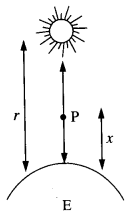Mass of Sun, M = 2 x 1030 kg
Mass of Earth, m = 6 x 1024 kg
Distance between Sun and Earth, r = 1.5 x 1011 m
Let at the point P,
the gravitational force on the rocket due to Earth = gravitational force on the rocket due to Sun
x = distance of the point P from the Earth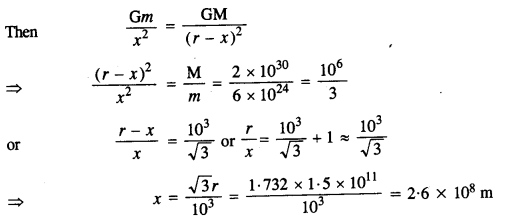Question 13.
How will you weigh the Sun, that is estimate its mass? You will need to know the period of one of its planets and the radius of the planetary orbit. The mean orbital radius of the Earth around the Sun is 1.5 x 108 km. Estimate the mass of the Sun.
Here radius of earth’s orbit (R + x) = 1.5 x 108 x 103 = 1.5 x 1011 m
Time period of sun, T = 365 days = 365 x 24 x 60 x 60 s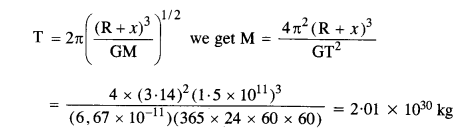Question 14.
A Saturn year is 29.5 times the Earth year. How far is Saturn from the Sun if the Earth is 1.50 x 108 km away from the Sun?
As we know the Kepler’s third law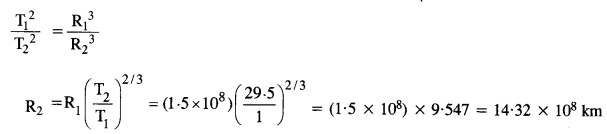Question 15.
A body weighs 63 N on the surface of the Earth. What is the gravitational force on it due to the Earth at a height equal to half the radius of the Earth?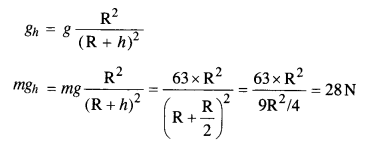Question 16.
Assuming the Earth to be a sphere of uniform mass density, how much would a body weigh halfway down to the center of the Earth if it weighed 250 N on the surface?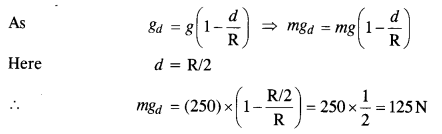Question 17.
A rocket is fired vertically with a speed of 5 km s-1 from the Earth’s surface. How far from the Earth does the rocket go before returning to the Earth? Mass of the earth = 6.0 x 1024 kg, mean radius of the Earth = 6.4 x 106 m ; G = 6.67 x 1011 N m2 kg-2.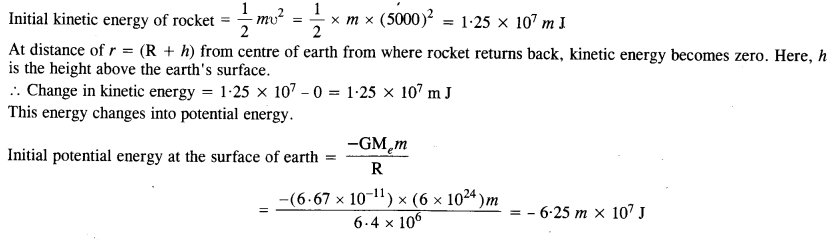Question 18.
The escape speed of a projectile on the Earth’s surface is 11.2 km s1. A body is projected out with thrice this speed. What is the speed of the body far away from the Earth? Ignore the presence of the Sun and the other planets.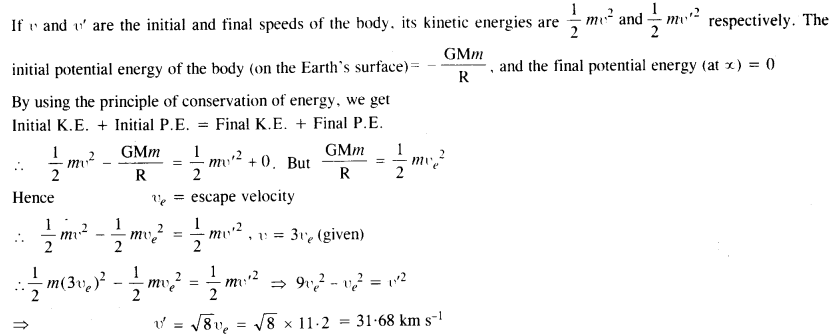Question 19.
A satellite orbits the Earth at a height of 400 km above the surface. How much energy must be expended to rocket the satellite out of the Earth’s gravitational influence? Mass of the satellite = 200 kg ; mass of the Earth = 6.0 x 1024 kg ; radius of the Earth = 6.4 x 106 m ; G = 6.67 x 1011 N m2 kg2
As we know, the total energy of a satellite in orbit,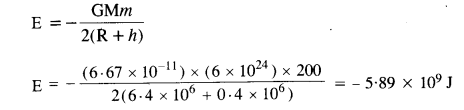Question 20.
Two stars each of one solar mass (= 2 x 1030 kg) are approaching each other for a head-on collision. When they are at a distance of 109 km, their speeds are negligible. What is the speed with which they collide? The radius of each star is 104 km. Assume the stars to remain undistorted until they collide. (Use the known value of G).
Here, M = 2 x 1030 kg ; R = 104 km = 107 m ; r = 109 km = 1012 mThis decrease in the potential energy of the system of two stars will appear as an increase in their K.E.
When the stars are at a distance of 109 km, their speeds are negligible and hence their initial K.E.s are also negligible. If υ is the speed with which the two stars collide, then increase in K.E. of the system of the two stars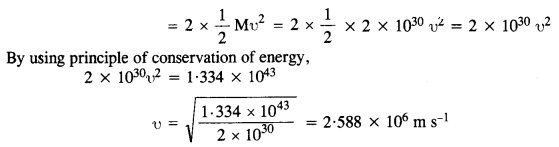Question 21.
Two heavy spheres each of mass 100 kg and radius 0.10 m are placed 1.0 m apart on a horizontal table. What is the gravitational Held and potential at the midpoint of the line joining the centers of the spheres? Is an object placed at that point in equilibrium? If so, is the equilibrium stable or unstable?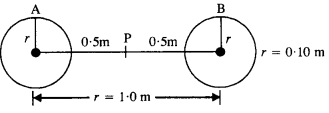The gravitational field at P due to sphere A is equal and opposite to the gravitational field at P due to sphere B.
Hence, the net gravitational field at P is zero.
Gravitational potential at P, V = VA + VB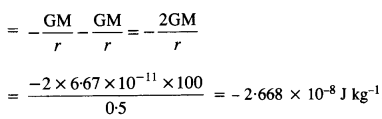Question 22.
As you have learned in the text, a geostationary satellite orbits the Earth at a height of nearly 36,000 km from the surface of the Earth. What is the potential due to Earth’s gravity at the site of this satellite? (Take the potential energy at infinity to be zero). Mass of the Earth = 6.0 x 1024 kg, radius = 6400 km.
Distance of satellite from the center of earth = R + r + x
= 6400 + 36000
= 42400 km
= 4.24 x 107 m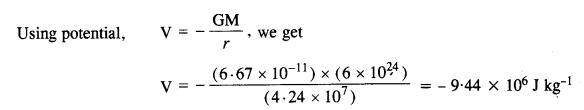Question 23.
A star 2.5 times the mass Of the Sun and collapsed to a size of 12 km rotates with a speed of 1.2 rev. per second. (Extremely compact stars of this kind are known as neutron stars. Certain observed stellar objects called pulsars are believed to belong to this category). Will an object placed on its equator remain stuck to its surface due to gravity? (mass of the Sun = 2 x 1030 kg).
The centripetal acceleration of the object placed at the equator of the star, ac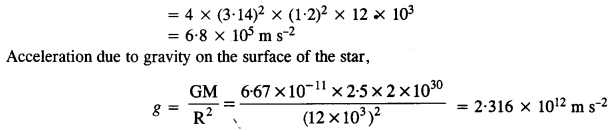Question 24.
A spaceship is stationed on Mars. How much energy must be expended on the spaceship to rocket it out of the solar system ? Mass of the spaceship = 1000 kg ; mass of the Sun = 2 x 1030 kg ; mass of Mars = 6.4 x 1023 kg ; radius of Mars = 3395 km ; radius of the orbit of Mars = 2.28 x 108 km ; G = 6.67 x 10-11 Nm2 kg-2.